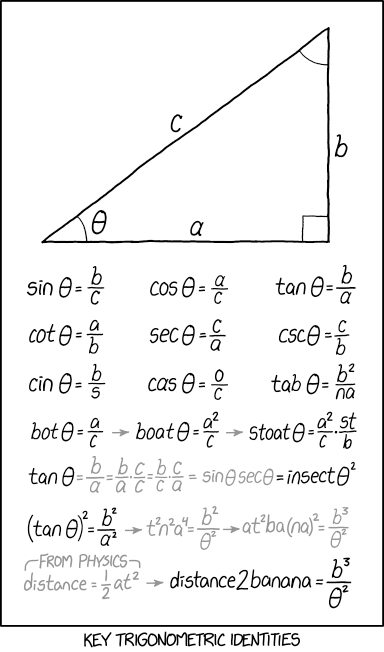← Back

November 9, 2018

# #2070: Trig Identities[Inside a single frame comic a right-angled triangle is shown. The shorter sides are labeled “a” and “b” and the hypotenuse has a “c”. All angles are marked: the right angle by a square and the two others by arcs. One arc (enclosed by “a” and “c”) is labeled by the Greek symbol theta (θ).]

[Supposed trigonometric functions of the marked angle θ are shown:]

sin θ = b/c

cos θ = a/c

tan θ = b/a

cot θ = a/b

sec θ = c/a

csc θ = c/b

cin θ = b/s

cas θ = o/c

tab θ = b²/n a

bot θ = a/c → boat θ = a²/c → stoat θ = a²/c · s t/b

tan θ ( = b/a = b/a · c/c = b/c · c/a = sin θ sec θ ) = insect θ²

(tan θ)² = b²/a² ( → t²n²a⁴ = b²/θ² → a t²b a(n a)² = b³/θ²

from physics: distance = 1/2 a t² → ) distance2banana = b³/θ²

[Caption below the frame:]

Key trigonometric identities